16 November 2018

Your PIC design will make more sense with text to identify different parts of your design.
This can be done by using the Nazca text() function. The text() function returns a cell with provided text of specified parameters. Below is a simple example of placing text in Nazca.

```# example created by Bright Photonics

import nazca as nd

nd.text('Nazca-Design').put()

nd.export_gds()```Text is flexible in height, layer, placement, alignment and font.
The default ‘nazca’ font is foundry friendly, as it has no sharp angles, no islands and no closed areas. If needed, the default ‘nazca’ font can be changed to the following other fonts: ‘liberation’, ‘cousine’ and ‘ocra’.

```# example created by Bright Photonics

import nazca as nd

message = 'Nazca-Design'
nd.text(text=message, height=30, layer=1, align='cc').put(0, 0)

f = nd.Font('cousine')
f.text(text=message, height=20, layer=2, align='cc').put(0, 40)

nd.export_gds()```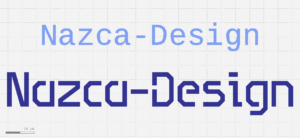Example below shows placing of text in Nazca using available parameters.
Make sure to use demofab_klayout_colors.lyp to view the mask in KLayout.

```# example created by Bright Photonics

import nazca as nd

for i in range(7):
T1 = nd.text(text=message, height=70*0.85**i, align='lb')
T1.put(0, -i*100)
T1.put(0, -1200+i*100)

T2 = nd.text(text=message, height=70*0.85**i, align='rb')
T2.put(4000, -i*100)
T2.put(4000, -1200+i*100)

nd.text('NAZCA', height=500, align='cc', layer=2).put(0.5*4000, -600)
nd.export_gds()```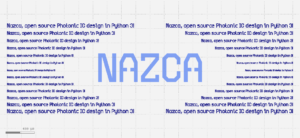## Related Tutorials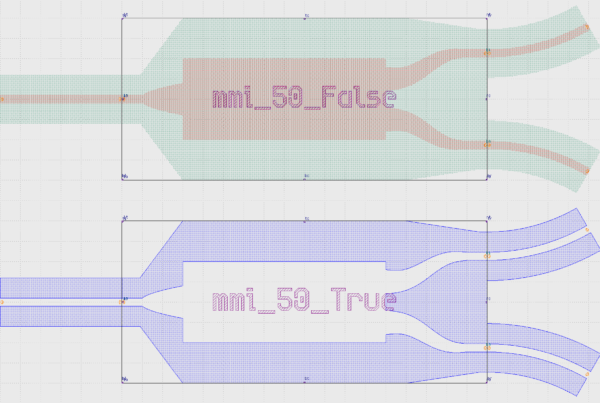In this example we show how to create an inverted MMI with custom interconnects.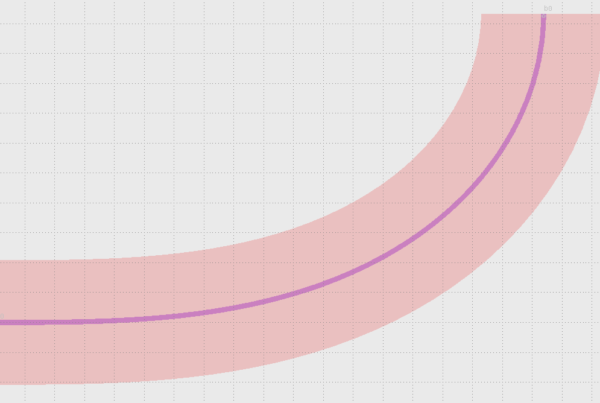In this example we show how to use Euler bends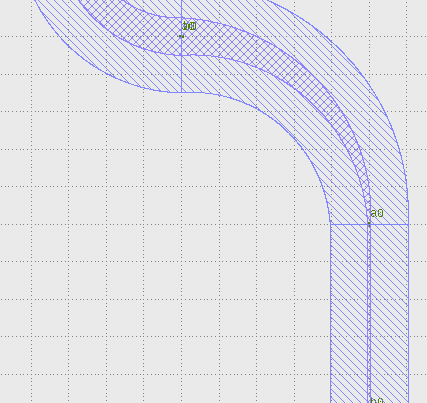In this example we show how to create a free form parametric curve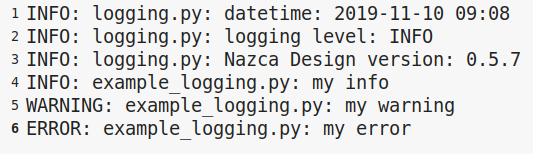In this example we show how to log your layout.